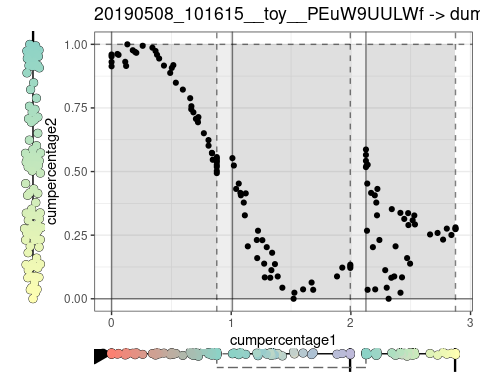Plotting a toy datasets using dynplot

2021-12-07

data(example_bifurcating)
trajectory <- example_bifurcating

Plotting the topology and cellular positions

If the topology is very simple (or should be represented in one dimension)

plot_onedim(trajectory)
## Coloring by milestone
## Using milestone_percentages from trajectory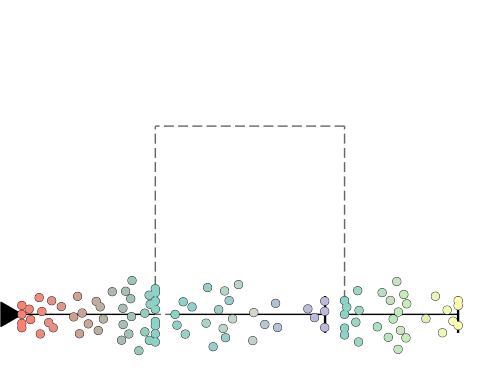If the topology is a tree

plot_dendro(trajectory)
## Coloring by milestone
## Using milestone_percentages from trajectory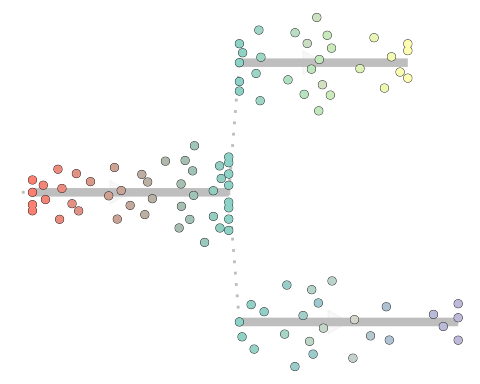If the topology is more complex

plot_graph(trajectory)
## Coloring by milestone
## Using milestone_percentages from trajectory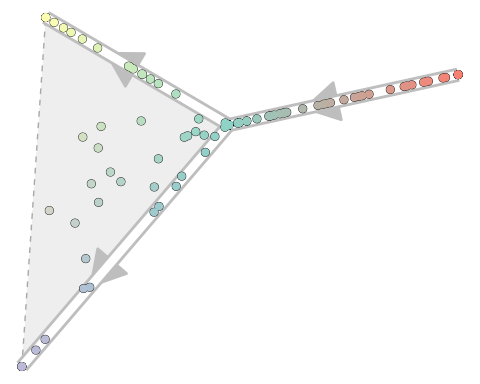plot_topology(trajectory, layout = "circle")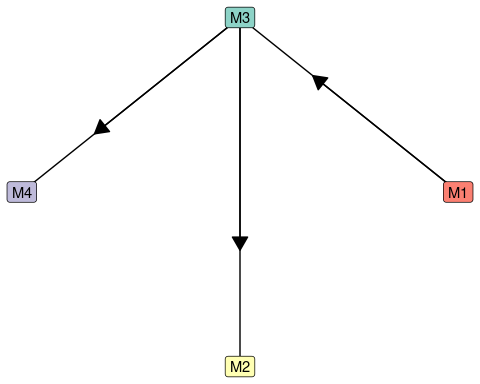plot_dimred(trajectory)
## Coloring by milestone
## Using milestone_percentages from trajectory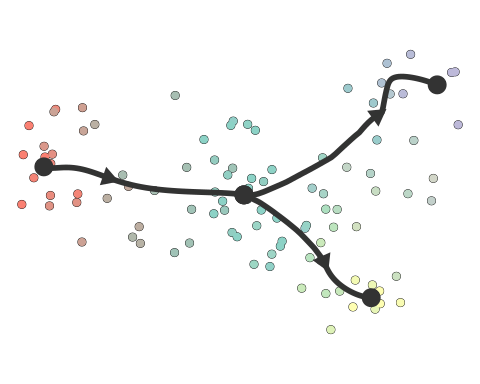plot_dimred(trajectory, color_trajectory = "nearest")
## Coloring by milestone
## Using milestone_percentages from trajectory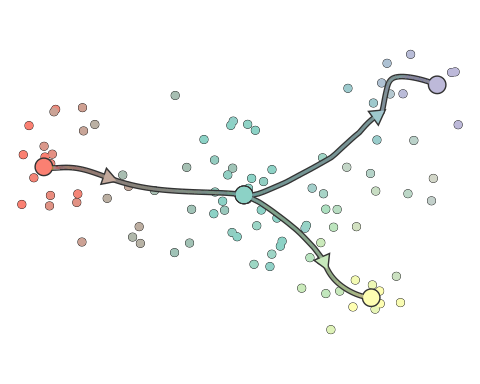Plotting a grouping or clustering

grouping <- trajectory$prior_information$groups_id
plot_onedim(trajectory, grouping = grouping)
## Coloring by grouping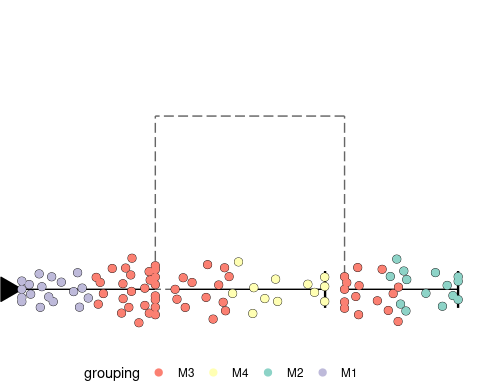plot_dendro(trajectory, grouping = grouping)
## Coloring by grouping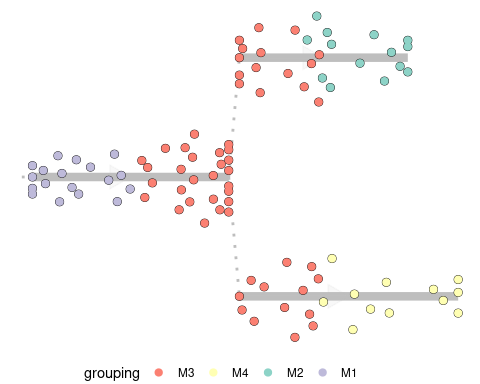plot_graph(trajectory, grouping = grouping)
## Coloring by grouping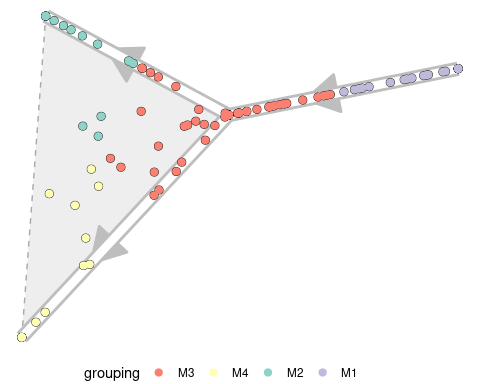plot_dimred(trajectory, grouping = grouping)
## Coloring by grouping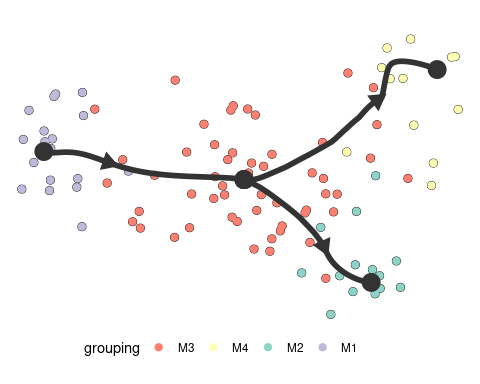feature_oi <- first(colnames(trajectory$counts)) plot_onedim(trajectory, feature_oi = feature_oi) ## Coloring by expression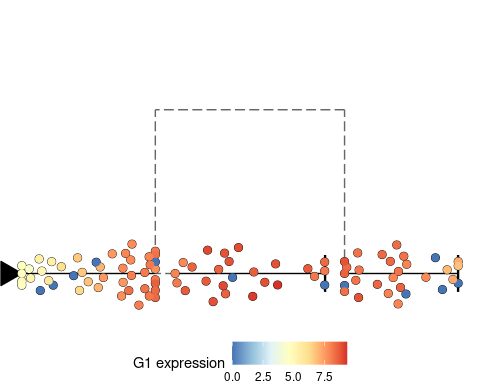plot_dendro(trajectory, feature_oi = feature_oi) ## Coloring by expression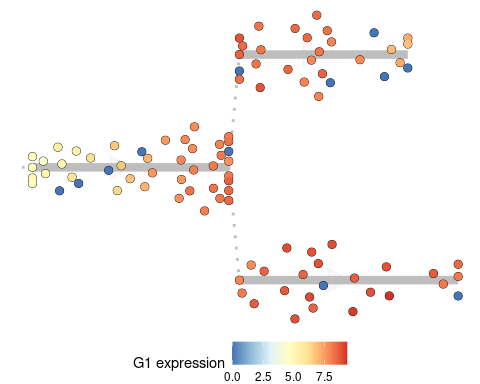plot_graph(trajectory, feature_oi = feature_oi) ## Coloring by expression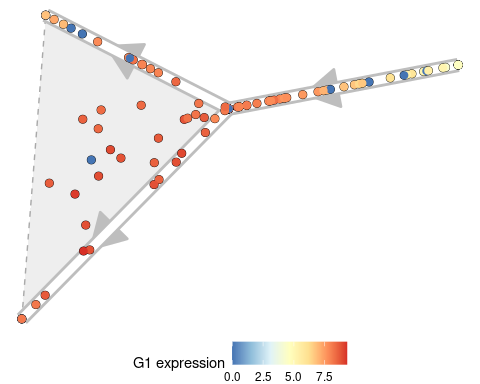plot_dimred(trajectory, feature_oi = feature_oi) ## Coloring by expression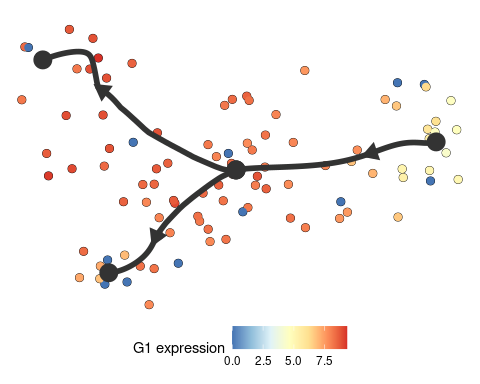Plotting expression of a lot of features plot_heatmap(trajectory) ## No features of interest provided, selecting the top 20 features automatically ## Using dynfeature for selecting the top 20 features ## Coloring by milestone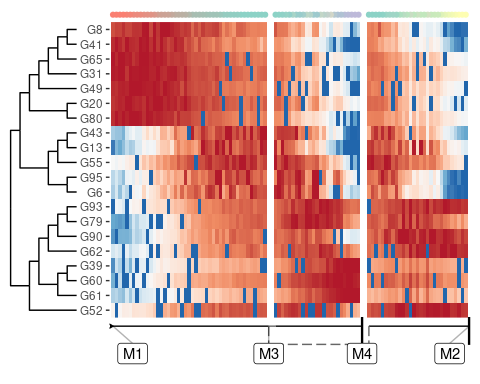Comparing trajectories pseudotime <- trajectory$counts %>% prcomp() %>% {.$x[, 1]} prediction <- dynwrap::wrap_data(id = "dummy_prediction", cell_ids = trajectory$cell_ids) %>%